## 两个有序数组 合并成一个有序数组

hphper 发布于 2015/03/11 20:53

\$a=array(2,5,7,9);

\$b=array(3,4,8,10,11);

array(2,3,4,5,7,8,9,10,11)

1```int i=0;int j=0;
int[] array = new int[a.length + b.length];
while(i<a.length && j<b.length){
array[i+j] = a[i]<b[j]?a[i++]:b[j++];
}
while(i<a.length){
array[i+j] = a[i++]
}
while(j<b.length){
array[i+j] = b[j++]
}```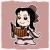0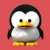\$a = array(2,5,7,9);
\$b = array(3,4,8,10,11);
\$c = array_merge(\$a, \$b);
sort(\$c);
print_r(\$c);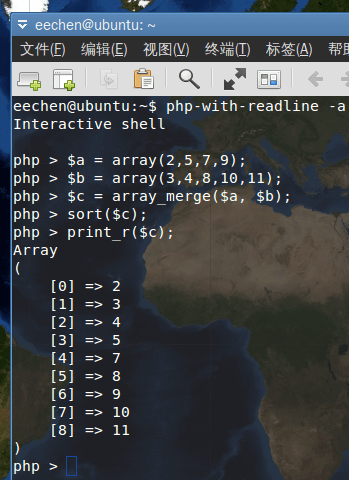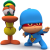0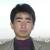php的数值（包括数组）操作，速度是很快的，基本不可能是性能瓶颈；这方面做优化，性价比不高。